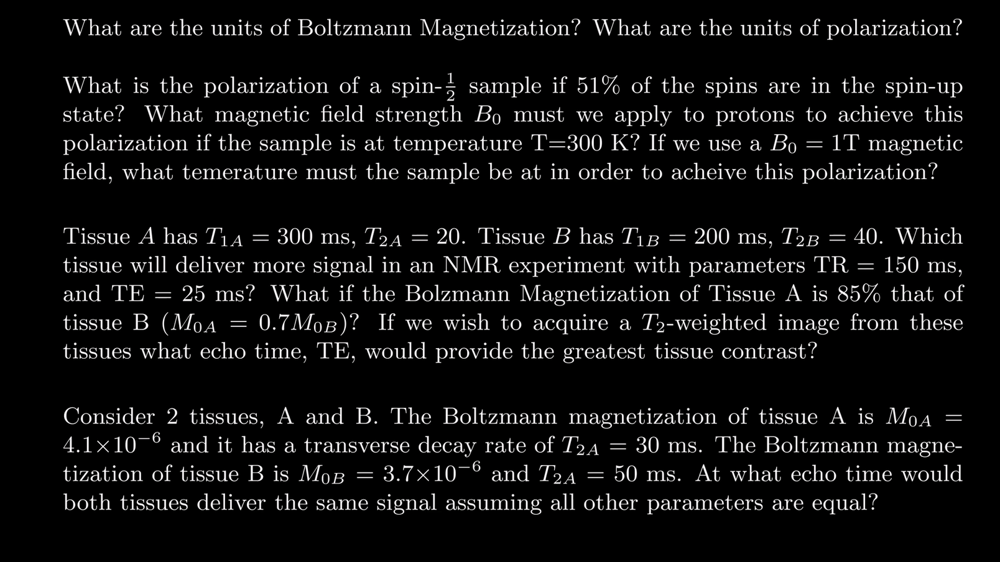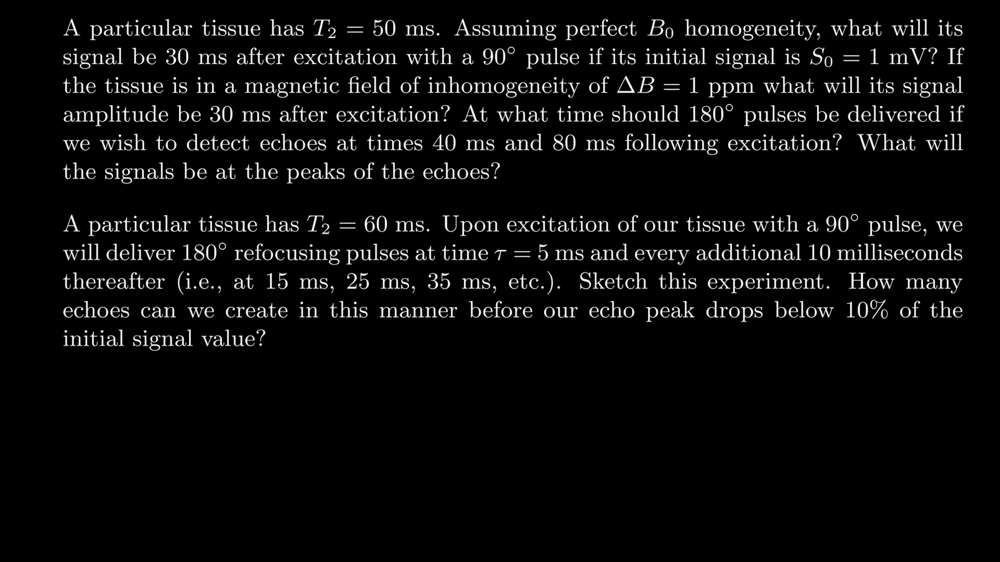By Frank Luo

This is my answers of the MRI Tutorial Videos How MRI Works - Part 2: The Spin Echo and How MRI Works - Part 3：Fourier Transform and K-Space .

## Part 2: The Spin Echo

### Questions#### Question 1：

1. The Boltzmann Magetization $M_0 = \frac{N {\gamma}^2 \hbar^2 B_0}{4 k T}$, then after elimination the units is $J/T$.
2. The Polarization is $P = \frac{\gamma \hbar B_0}{2kT}$, then after elimination we can get that $P$ is a special number depends on the material, no SI units.

#### Question 2：

1. The polarization is $P = \frac{51-49}{100} = 0.02$ .
2. The magnet field strength should be $B_0 = \frac{0.02}{0.0000034} \approx 5882T$ .
3. The temperature should be $T = \frac{300 \times 0.0000034}{0.02} = 0.051K$.

#### Question 3：

1. Since the Boltzmann Magetization Equation is $M = M_0(1- e^{-\frac{t}{T_1}}) e^{-\frac{t}{T_2}}$ , so we can calculate the signal.

The signal of Tissue $A$ : $M_A = M_0(1- e^{-\frac{150}{300}}) e^{-\frac{12.5}{20}} = 0.21$ .
The signal of Tissue $B$ : $M_B = M_0(1- e^{-\frac{150}{200}}) e^{-\frac{12.5}{40}} = 0.38$ .

Surely Tissue $B$ will deliver more signal.

1. We have calculated that Tissue $B$ will deliver more signal if both Tissue $A$ and $B$ has the same Boltzmann Magetization.

If Tissue $A$ is $85\%$ of Tissue $B$, then the Tissue $A$ signal will become lesser, so Tissue $B$ deliver more signal.

1. Let function $f(t) = M_{0A}(1- e^{-\frac{TR}{T_{1A}}})e^{-\frac{t}{T_{2A}}} - M_{0B}(1- e^{-\frac{TR}{T_{1B}}})e^{-\frac{t}{T_{2B}}}$ reprent the signal of time $t$.

Consider the function: $f(t) = (1 - e^{-\frac{150}{200}}) e^{-\frac{t}{40}} - (1- e^{-\frac{150}{300}}) e^{-\frac{t}{20}}$ reaches its PEAK at about $t = 16$, so the $TE$ should be $TE = 32ms$.

#### Question 4：

If both tissues deliver the SAME signal, which means $M_{0A} e^{-\frac{t}{T_{2A}}} = M_{0B}e^{-\frac{t}{T_{2B}}}$.

Put the data in, then we can get $4.1e^{-\frac{t}{30}} - 3.7e^{-\frac{t}{50}} = 0$, solve it and get $t \approx 7.7ms$.

So the echo time is: $TE = 2 \times t \approx 15.4ms$.

#### Question 5：

1. $e^{-\frac{t}{T_2}}S_0 = e^{-\frac{30}{50}} \approx 0.55$, so the signal is $0.55mV$.

2. If the magetic field of inhomogeneity of $\Delta B = 1$ ppm, the signal can be calculated by such equation:

$S(t) = S_0 e^{-\frac{t}{T_2}} e^{- \gamma \Delta B t}$

Put the data in, we can $e^{-\frac{30}{50}} e^{-1 \times 267 \times 0.000003} \approx 0.43$, so the signal amplitude is $0.43$.

1. We should delivered the 180° pulses at times 20ms and times 40ms if we wish to detect echoes at times 40ms and 80ms.

The signal will be $e^{-\frac{40}{50}} \approx 0.45$ at times 40ms and $e^{-\frac{80}{50}} \approx 0.20$ at times 80ms.

#### Question 6：

From the equation $M = M_0 e^{-\frac{t}{T_2}}$, then we can solve $e^{-\frac{t}{60}} \le 0.1$, the answer is $t \approx 138.55ms$.

Therefore, we can get the echoes at times 10ms, 30ms, 50ms, 70ms, 90ms, 110ms, 130ms, so we can get $7$ echoes.

## Part 3: Fourier Transform and K-Space

### QuestionsThe amplitude of the $\textit{FFT}$ result is $15$. We need to times $\frac{2}{N}$ to get the correct answer.
1. Both the positive and negative frequency contribute the answer, but we only use the positive, so have to multiply $2$.
2. Each operation we have to sum once, so we need the result to multiply $\frac{1}{N}$ to get the final answer.